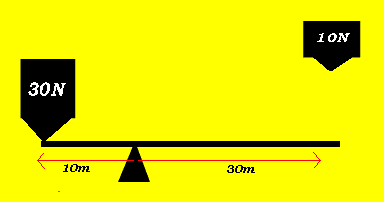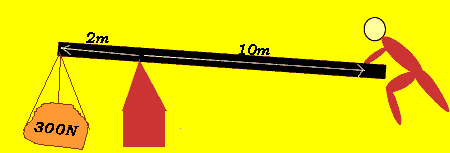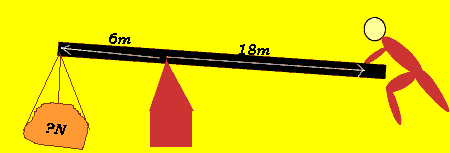Levers Moment When a lever is in equilibrium the two opposing forces just balance each other. The moments on both sides of the fulcrum are equal. Take the case on the right. The 30N force 10m away from the fulcrum is just balanced by the 10N force 30m from the fulcrum. The moments are equal according to the expression below 30N X 10m = 10N X 30mWhat is the minimum force that is required to lift the load with a force acting on it of 300N? Firstly the moments must be equal on both sides of the fulcrum at the point where the load force just balances the effort force.. 2m X 300N = 10m X effort => effort = 60N. So the minimum force required to just lift the load is 60N.What is the maximum force that can be lifted with a force of 300N if the lever on the right is used? Firstly the moments must be equal on both sides of the fulcrum at the point where the load force just balances the effort force. 6m X load = 18m X 300N => load = 900N. So the maximum force that can be opposed with a force of 300N is 900N.Home